# Pascal geometry

(diff) ← Older revision | Latest revision (diff) | Newer revision → (diff)

A plane geometry constructed over a field (a commutative skew-field). The name is derived from the fact that in this geometry the configuration of the Pappus–Pascal proposition holds: If points 1, 3, 5 and 2, 4, 6 lie on two straight lines (are collinear), then the points of intersection of the pairs of lines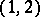and,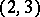and,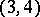and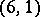— say, 9, 7 and 8 — also lie on a single line for every choice of three points each on any two straight lines (see Fig.).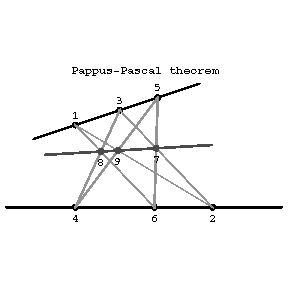Figure: p071760a

The most important special case of this proposition (in an affine plane) asserts that if the lineis parallel to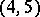andis parallel to, thenandare also parallel.

Pascal geometries in the plane can be constructed over infinite or finite fields and accordingly the plane is called an infinite or finite Pascal plane.

The important role of Pascal's proposition in the construction of geometric systems over an infinite field was first investigated by D. Hilbert in , who established that it can be proved for various collections of axioms from the axiom system of Euclidean geometry. He also showed that the Pascal theorem in an infinite plane can be inferred from the plane axioms of incidence, order, congruence, parallelism, and continuity, and that without the axiom of continuity in this case Pascal's theorem cannot be proved.

Relying on Hilbert's axiom system in space, Pascal's theorem can be proved without the congruence axioms, but necessarily with an application of the continuity axiom. (The omission of the latter in an infinite plane leads to non-Pascalean geometry.) The possibility of proving Pascal's theorem is similar in a certain sense to that of proving the Desargues assumption with the use of spatial axioms. However, in the proof of Pascal's theorem the particular role of Archimedes' axiom of continuity in infinite planes manifests itself (see Non-Archimedean geometry).

The Pappus–Pascal proposition holds projectively in a plane if and only if in all natural skew-fields of this plane multiplication is commutative; in other words: the natural skew-field of every Pascal plane is a field and, conversely, a plane constructed over a field has a Pascal geometry. Therefore the latter is sometimes called a geometry with commutative multiplication. Thus, in a Pascal plane the configuration of the Pappus–Pascal proposition has an algebraic interpretation: it expresses the commutativity of multiplication in the set over which the plane is constructed.

In any projective plane the Pappus–Pascal theorem implies Desargues' theorem.

A finite Pascal plane, like a finite projective plane, exists if and only if the number of points on each line of the plane is, whereis a prime anda natural number.

Since every finite alternative skew-field is a field, in a finite plane Desargues' theorem implies the Pappus–Pascal theorem, and the latter is a consequence of the so-called little Desargues theorem. However, there exist finite projective non-Pascalean planes. A Pascal plane is isomorphic to its dual.

The merit of Pascal geometry lies in its role in research on the independence of axiom systems, in particular, Hilbert's axiom system of Euclidean geometry. In the construction of an infinite plane on the basis of the groups of axioms of incidence, order and parallelism, the Pappus–Pascal proposition must be regarded as an additional axiom. On the other hand, by means of a combination of finitely many Pappus–Pascal configurations one can solve problems on constructions in which only the notion of incidence is used.

How to Cite This Entry:
Pascal geometry. Encyclopedia of Mathematics. URL: http://encyclopediaofmath.org/index.php?title=Pascal_geometry&oldid=11435
This article was adapted from an original article by L.A. Sidorov (originator), which appeared in Encyclopedia of Mathematics - ISBN 1402006098. See original article